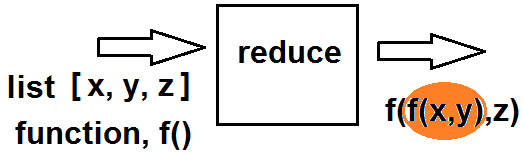﻿ How to Create a Reduce Function in Python ﻿# How to Create a Reduce Function in PythonIn this article, we go over how to create a reduce function in Python

Before we do that, let's first go over what a reduce function is?

A reduce function is basically a function that performs the same operation to an items of a sequence, using the result of each operation as the first parameter of the next operation. A reduce function, in the end, returns an item, not a list, unlike map() and filter() functions.

So a great example of thinking about a reduce() function is thinking about computing the factorial of a number.

Let's say we want to compute the factorial of 5, so 5!

To do this, we take 5 and multiply it by 4; the product of 5x4=20. We then take 20 and multiply it by 3 to get 60. We then take 60 and multiply it by 2 to get 120. We then take 120 and multiply it by 1 to get 120.

Notice we do 5x4=20. We then take the product and bring it as the first parameter in the next operation, which is 20x3=60. Then 60 becomes the first parameter of the next operation, which is 60x2= 120.

This is how a reduce() function works in Python.

The following diagram below helps to illustrate the reduce function in Python.As you can see from the diagram, a list is passed into this reduce function. An operation is performed first on the first 2 functions. The result of this operation is then passed as the first parameter of the next function. And this goes on until the function operates on all elements of the list.

So into a reduce() function in Python, you pass in 2 parameters, just like with a map() function or the filter() function. The first parameter is the function (the operation to carry out) and the second parameter is the list (or iterable object).

So this is a reduce function in Python.

In the following code shown below, we create a reduce() function that functions as a factorial calculator.

The first thing we have to do is import the reduce() function. We do this by the line, from functools import reduce

After we create a list. This list is composed of 5 numbers: 5,4,3,2,1

We then create a reduce() function and assign it to the factorial variable.

Inside of this reduce() function, the function we pass in is a lambda function. Therefore, we don't have to create some external function. We create a lambda function on the spot. We then pass in the second parameter, which is our iterable object, the list.

We then print out the factorial variable.

We then get as output, 120

This is because, 5!= 5x4x3x2x1= 120

And this is how to use reduce functions in Python.

Related Resources

How to Randomly Select From or Shuffle a List in Python